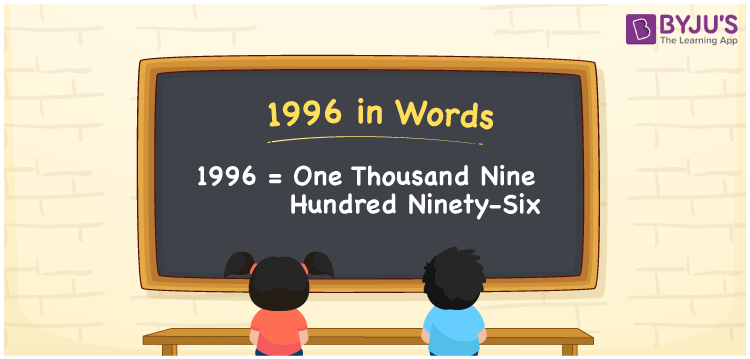# 1996 in Words

1996 in words is written as “One Thousand Nine Hundred ninety-six”. In Maths, 1996 is a counting number that expresses a quantity. To write a cheque for Rs.1996, we can write in words as Rupees One Thousand Nine Hundred ninety-six only. Learn more about Numbers In Words and writing the number names in English at BYJU’S.

 1996 in Words One Thousand Nine Hundred ninety-six One Thousand Nine Hundred ninety-six in Numbers 1996

## 1996 in English Words

See the chart below to learn the number name of 1996 in words.## How to Write 1996 in Words?

The number 1996 in words can be written using a place value system, where we can identify the position of each digit in the original number. Since 1996 is a four-digit number, thus, we can represent each digit as:

 Thousands Hundreds Tens Ones 1 9 9 6

From the above table,

1 → Thousand place

9 → Hundred place

9 → Tens place

6 → Ones place

Hence, when we read the number from right to left, it is given by One Thousand Nine Hundred ninety-six.

### Expanded Form of 1996

We can write the expanded form as:

1 x Thousand + 9 × Hundred + 9 × Ten + 6 × One

= 1 x 1000 + 9 × 100 + 9 × 10 + 6 × 1

= 1000 + 900 + 90 + 6

= One Thousand Nine Hundred ninety-six

1996 is a whole number that is succeeded by 1995 and preceded by 1997. Learn more about the number 1996 below:

• 1996 in Words – One Thousand Nine Hundred ninety-six
• Is 1996 an odd number? – No
• Is 1996 an even number? – Yes
• Is 1996 a perfect square number? – No
• Is 1996 a perfect cube number? – No
• Is 1996 a prime number? – No
• Is 1996 a composite number? – Yes

## Frequently Asked Questions on 1996 in words

Q1

### What is 1996 in words?

1996 in words is given by One Thousand Nine Hundred ninety-six.
Q2

### What is the rule to write 1996 in words?

To write the 1996 in words, we should use place value rule.

Thousands – 1, Hundreds – 9, Tens – 9, Ones – 6

Thus, we can name the number 1996 as One Thousand Nine Hundred ninety-six in words.

Q3

### What is the value of 1900 + 96 in words?

1900 + 96 = 1996 , i.e.,One Thousand Nine Hundred ninety-six.